Question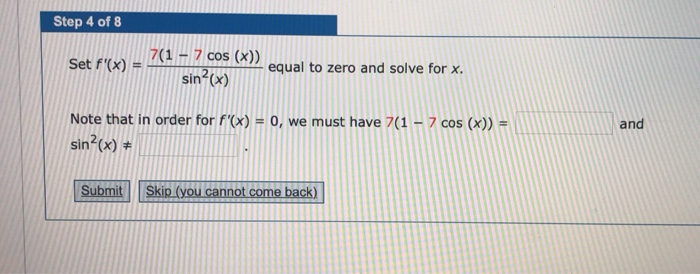We need at least 10 more requests to produce the answer.

0 / 10 have requested this problem solution

The more requests, the faster the answer.

All students who have requested the answer will be notified once they are available.

#### Earn Coins

Coins can be redeemed for fabulous gifts.

Similar Homework Help Questions
• ### Step 2 Compare y = a sin bx and y = -7 sin x and be...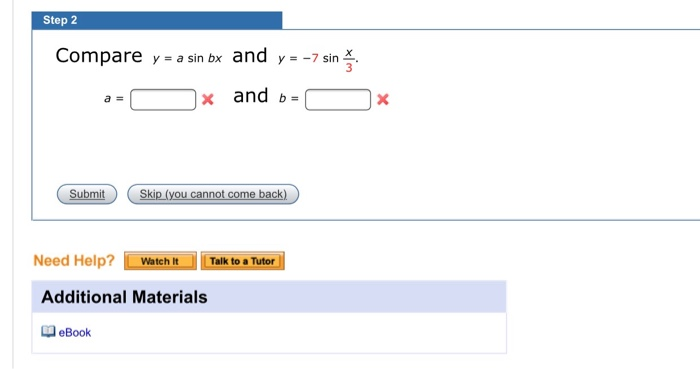Step 2 Compare y = a sin bx and y = -7 sin x and be X Submit Skip (you cannot come back) Need Help? Watch It Talk to a Tutor Additional Materials eBook

• ### Step 2 For F(x, y, 2) = 8exy sin(2) ј+ Зy tan- n (3) k, we...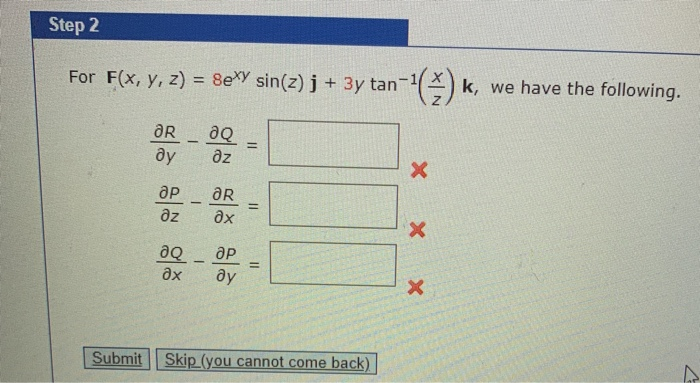Step 2 For F(x, y, 2) = 8exy sin(2) ј+ Зy tan- n (3) k, we have the following. дR - дQ ду дz E др — aR дх дz X X X дQ дх ӘР ду Submit Skip (you cannot come back)

• ### Tutorial Exercise Find the indicated derivative. If f(x) = x + 5, find f'(x). Step 1...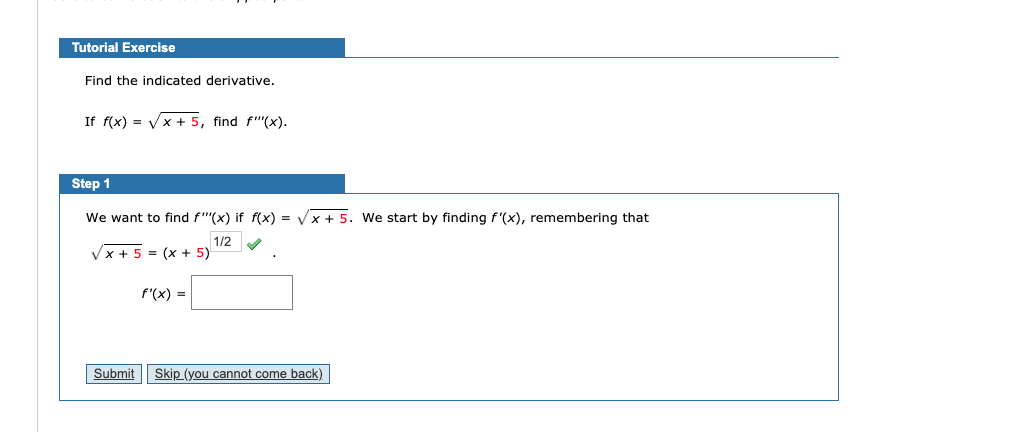Tutorial Exercise Find the indicated derivative. If f(x) = x + 5, find f'(x). Step 1 We want to find f'(x) if f(x) = x + 5. We start by finding f'(x), remembering that Vx+ 5 = (x + 5) 112 v. f(x) = Submit Skip (you cannot come back)

• ### Step 7 of 9 Find lim f(x) by looking at the right-hand and left-hand limits as...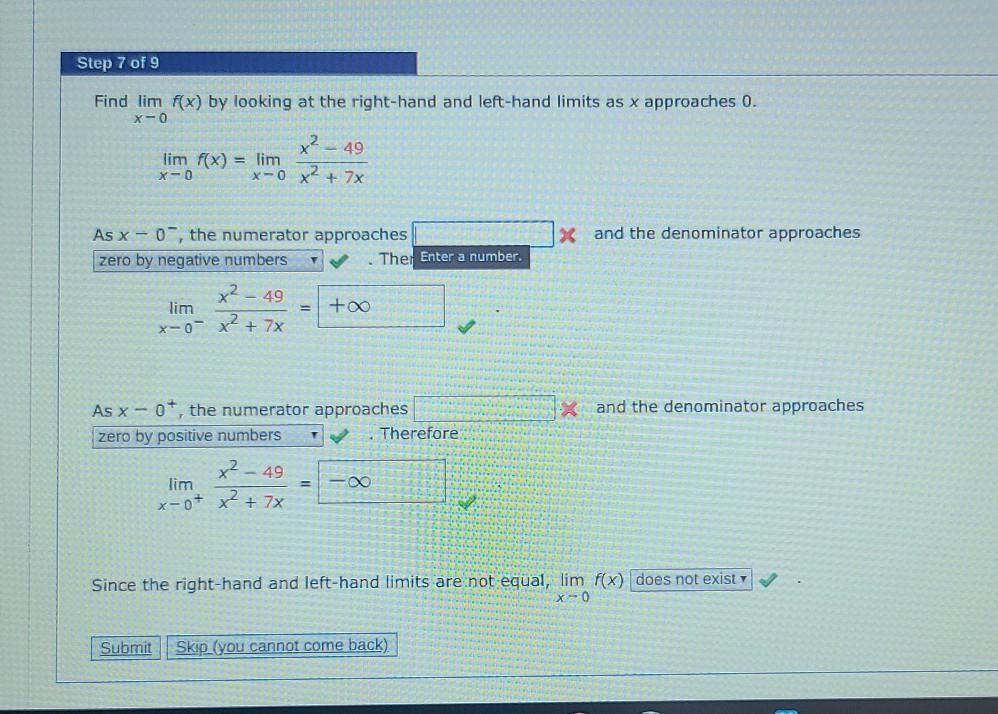Step 7 of 9 Find lim f(x) by looking at the right-hand and left-hand limits as x approaches 0. X-O x? - 49 lim f(x) = lim x-0 xto x² + 7x X and the denominator approaches As X - 0 , the numerator approaches zero by negative numbers Ther Enter a number. x2 - 49 +00 lim x-o- x² + 7x X and the denominator approaches As x - 07, the numerator approaches zero by positive numbers Therefore x²...

• ### Exercise (6) COS(10) Step 1 Recall that cosine has period 2. We must first recognize that...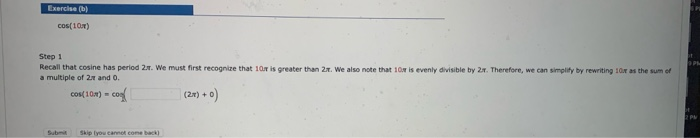Exercise (6) COS(10) Step 1 Recall that cosine has period 2. We must first recognize that our is greater than 2t. We also note that 10 is evenly divisible by 2.7. Therefore, we can simplify by rewriting as the sum of a multiple of 2.2 and 0. cos(10) con + Sub Skip you cannot come back)

• ### Given the integral below, do the following. 2 cos(x2) dx Exercise (a) Find the approximations T4...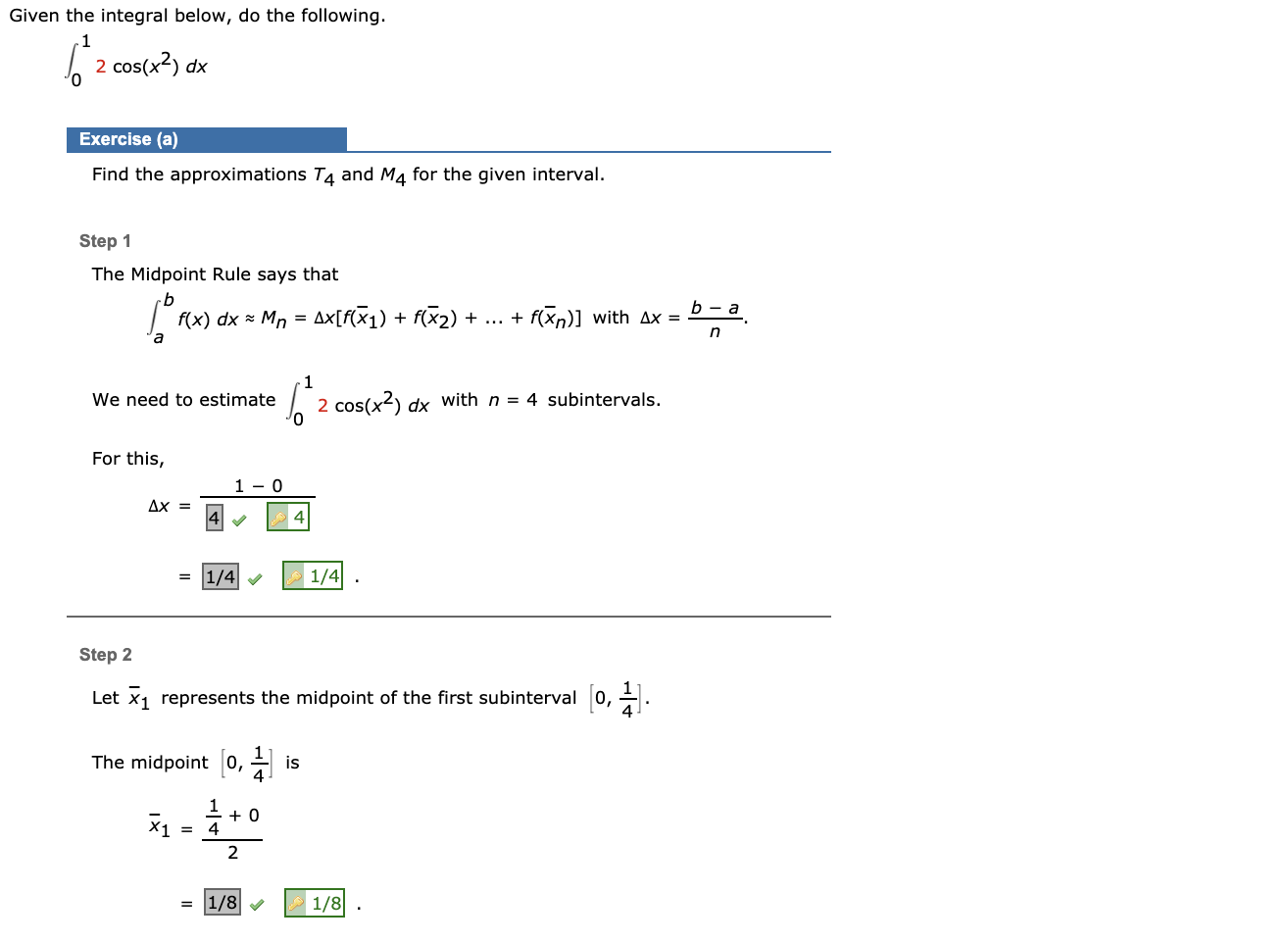Given the integral below, do the following. 2 cos(x2) dx Exercise (a) Find the approximations T4 and M4 for the given interval. Step 1 The Midpoint Rule says that b f(x) dx = Mn Ax[f(+1) + f(22) + ... + f(n)] with ax = . b - a + n a 1 We need to estimate 6 2 cos(x2) dx with n = 4 subintervals. For this, 1 - 0 Ax = 4 = 1/4 1/4 Step 2 Let žų...

• ### Step Now we can see that b-14b - 14b 1e-14 196 1 15 -1 lim 0-...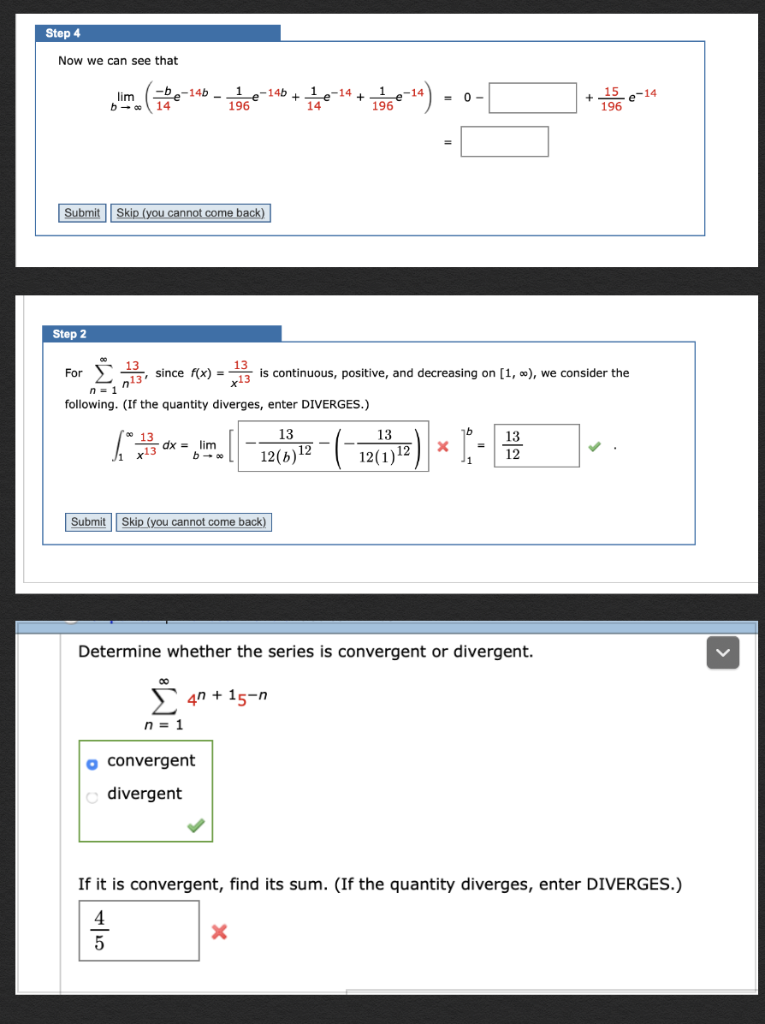Step Now we can see that b-14b - 14b 1e-14 196 1 15 -1 lim 0- 14- 196 14 Submit Skip (you cannot come back) Step 2 13 is continuous, positive, and decreasing on [1, o), we consider the since f(x)= For al3 13 n= 1 following. (If the quantity diverges, enter DIVERGES.) 13 13 13 13 dx=lim - 12(b)12 12(112 x13 12 b Submit Skip (you cannot come back) Determine whether the series is convergent or divergent. 4n+15-n n=...

• ### Step 1 Differentiate f(x) = -5x2 + 20x + 4 with respect to x. f'(x) =...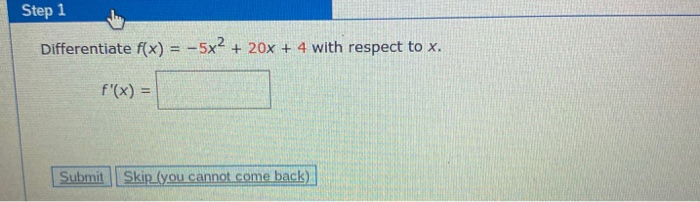Step 1 Differentiate f(x) = -5x2 + 20x + 4 with respect to x. f'(x) = Submit Skip_(you cannot come back)

• ### 2s 1 x 5 (s - 3)(s + 4) Step 3 Partial fraction decomposition can now...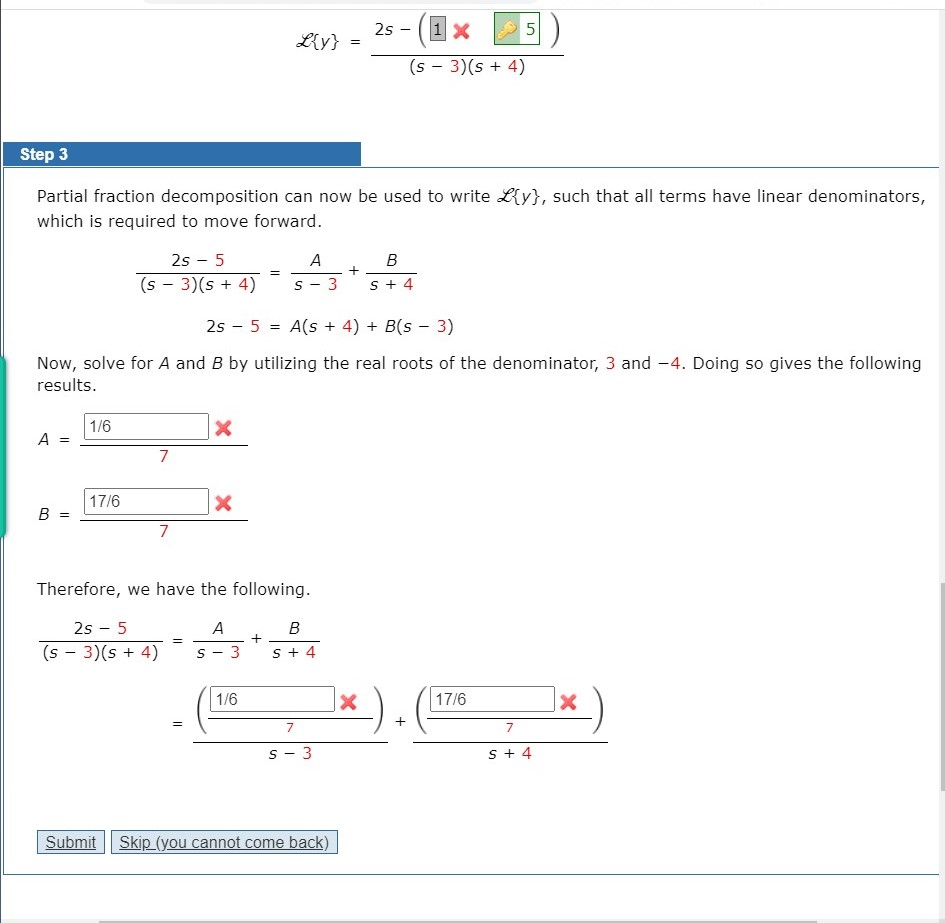2s 1 x 5 (s - 3)(s + 4) Step 3 Partial fraction decomposition can now be used to write L{y}, such that all terms have linear denominators, which is required to move forward. А B + 2s - 5 (s - 3)(8 + 4) S 3 S + 4 = 2s - 5 Als + 4) + B(5 - 3) Now, solve for A and B by utilizing the real roots of the denominator, 3 and - 4. Doing...

• ### Convert the polar equation to rectangular form and sketch its graph. r = 7 cot(0) csc(O)...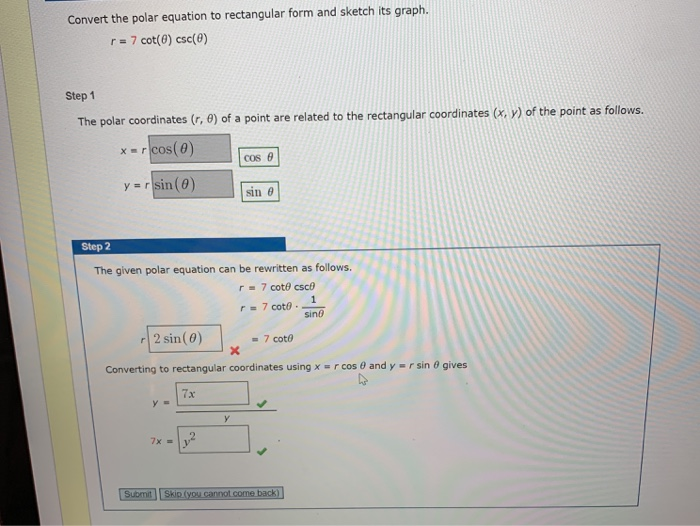Convert the polar equation to rectangular form and sketch its graph. r = 7 cot(0) csc(O) Step 1 The polar coordinates (r, e) of a point are related to the rectangular coordinates (x, y) of the point as follows. x=rcos(0) cos y = r sin(0) sin e Step 2 The given polar equation can be rewritten as follows. r 7 cote csco 1 r = 7 coto sino 2 sin(0) = 7 coto Converting to rectangular coordinates using x =...